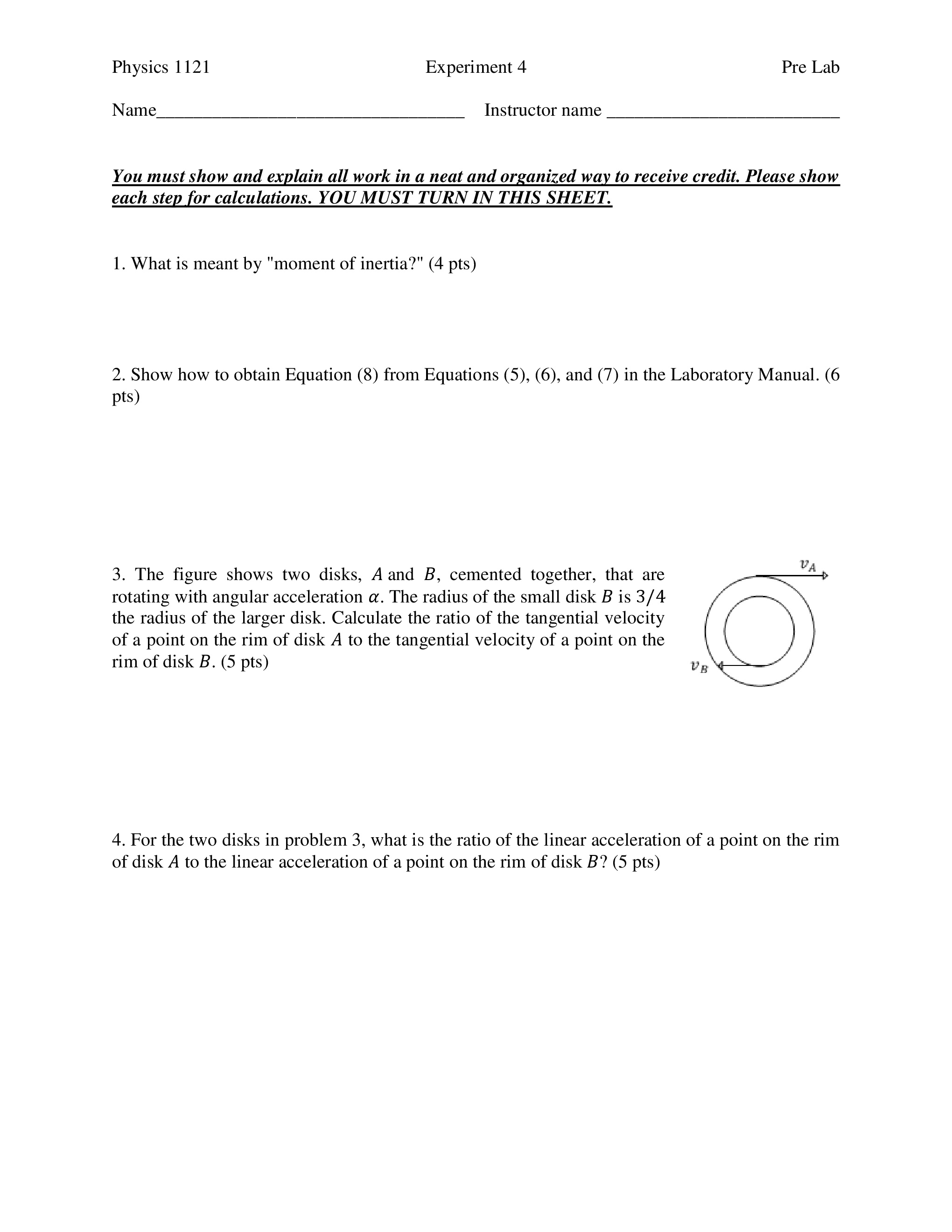# Physics 1121 Experiment 4 Pre Lab Name Instructor name You must show and explain all work in a neat and organized way to receive credit. Please show each step for calculations. YOU MUST TURN IN THIS SHEET. 1. What is meant by "moment of inertia?" (4 pts) 2. Show how to obtain Equation (8) from Equations (5), (6), and (7) in the Laboratory Manual. (6 pts) LV 3. The figure shows two disks, A and B, cemented together, that are rotating with angular acceleration α. The radius of the small disk B is 3/4 the radius of the larger disk. Calculate the ratio of the tangential velocity of a point on the rim of disk A to the tangential velocity of a point on the rim of disk B. (5 pts) LV 4. For the two disks in problem 3, what is the ratio of the linear acceleration of a point on the rim of disk A to the linear acceleration of a point on the rim of disk B? (5 pts)

Question

Numbers 3 and 4 on attached image.

3)The figure shows two disks, ? and ?, cemented together, that are
rotating with angular acceleration ?. The radius of the small disk ? is 3/4
the radius of the larger disk. Calculate the ratio of the tangential velocity
of a point on the rim of disk ? to the tangential velocity of a point on the
rim of disk ?.

4)For the two disks in problem 3, what is the ratio of the linear acceleration of a point on the rim
of disk ? to the linear acceleration of a point on the rim of disk ??help_outlineImage TranscriptionclosePhysics 1121 Experiment 4 Pre Lab Name Instructor name You must show and explain all work in a neat and organized way to receive credit. Please show each step for calculations. YOU MUST TURN IN THIS SHEET. 1. What is meant by "moment of inertia?" (4 pts) 2. Show how to obtain Equation (8) from Equations (5), (6), and (7) in the Laboratory Manual. (6 pts) LV 3. The figure shows two disks, A and B, cemented together, that are rotating with angular acceleration α. The radius of the small disk B is 3/4 the radius of the larger disk. Calculate the ratio of the tangential velocity of a point on the rim of disk A to the tangential velocity of a point on the rim of disk B. (5 pts) LV 4. For the two disks in problem 3, what is the ratio of the linear acceleration of a point on the rim of disk A to the linear acceleration of a point on the rim of disk B? (5 pts) fullscreen

### Want to see this answer and more?

Experts are waiting 24/7 to provide step-by-step solutions in as fast as 30 minutes!*